Question

# From the following data, C(graphite) + O2(g) → CO2(g) AHrxn = -393.5 kJ/mol H2(g) + O2(g)...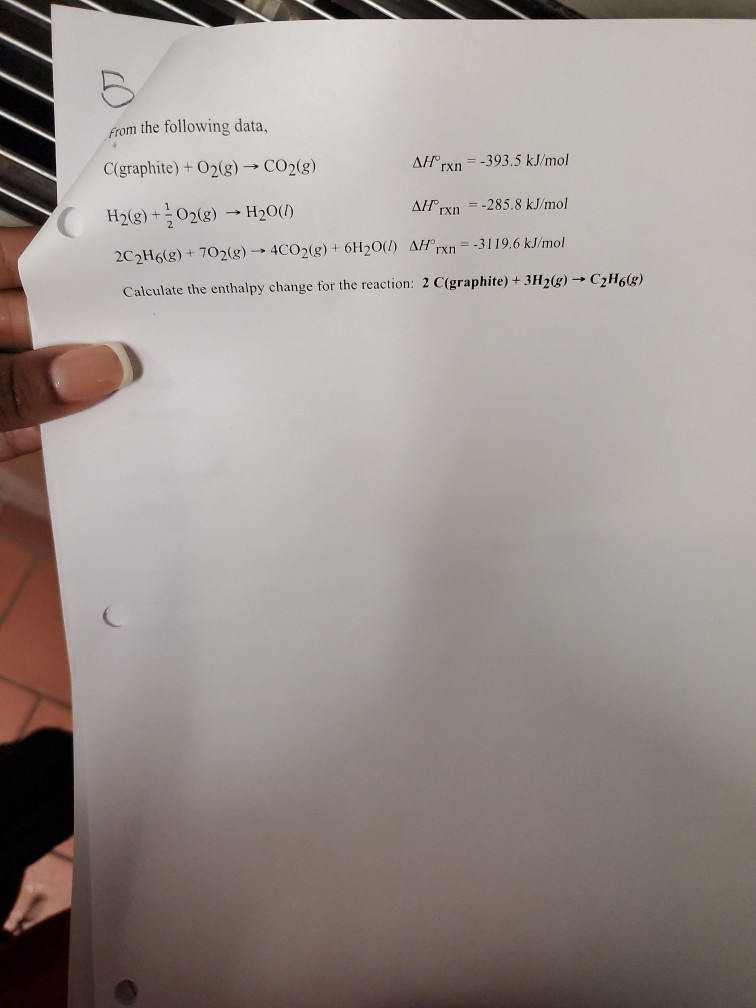From the following data, C(graphite) + O2(g) → CO2(g) AHrxn = -393.5 kJ/mol H2(g) + O2(g) → H200) AH"rxn = -285.8 kJ/mol 2C2H6(g) + 702(g) -> 4CO2(g) + 6H2O(l) Arxn=-3119.6 kJ/mol Calculate the enthalpy change for the reaction: 2 C(graphite) + 3H2(g) + C2H668)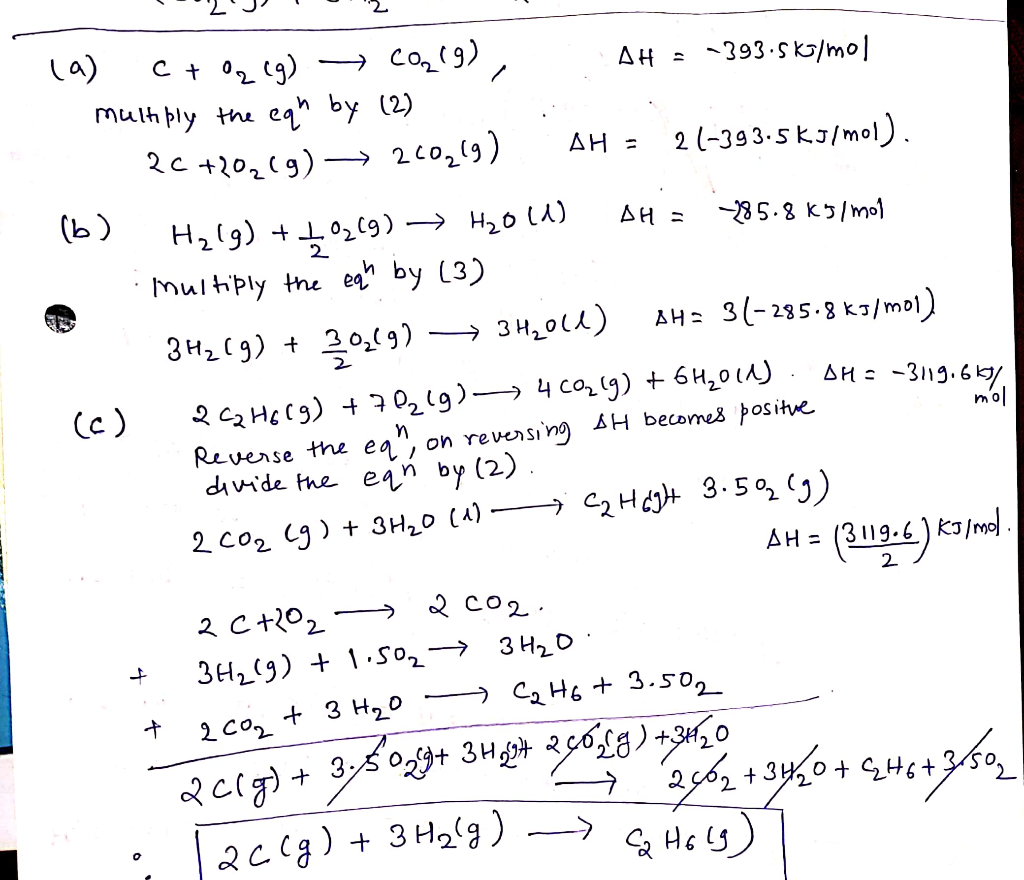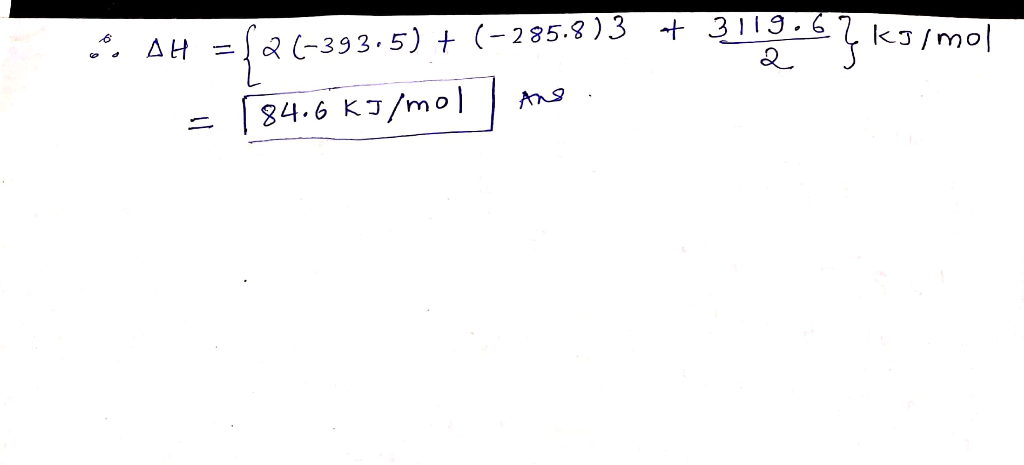Answer will be -84.6 kJ/mol not positive

#### Earn Coins

Coins can be redeemed for fabulous gifts.

Similar Homework Help Questions
• ### Enter your answer in the provided box From the following data, C(graphite) + O2(0)+ CO2(g) An°...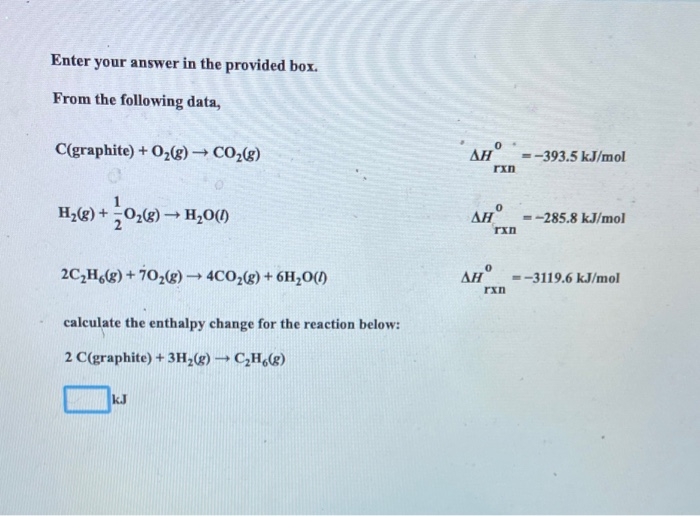Enter your answer in the provided box From the following data, C(graphite) + O2(0)+ CO2(g) An° . =-393.5 kJ/mol rxn Hy(@) +0,6) H200 AH =-285.8 kJ/mol rxn 2C2H6(8) + 1026) →40026) + 6H20(1) AH =-3119.6 kJ/mol rxn calculate the enthalpy change for the reaction below: 2 C(graphite) + 3H2(g) → CH()

• ### hess's law

from the folling data:C(graphite)+O2-->CO2(g) ?H rxn = -393.5 kJ/molH2(g) +1/2 O2(g) --> H2O(l) ?H rxn = -285.8 kJ/mol2C2H6(g) + 7O2(g) --> 4CO2 (g) + 6H2O(l) ?H rxn = -3119.6 kJ/molcalculate the enthalpy for the reaction2C(graphite) + 3H2(g)-->C2H6(g)

• ### Please explain Data: C(graphite) + O2(g) => CO2(g) AH = -393.5 kJ H2(g) + 1/2O2(g) =>...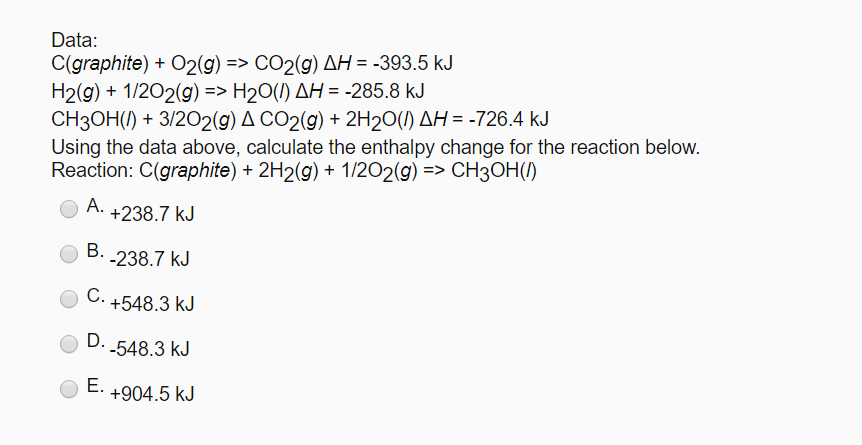Please explain Data: C(graphite) + O2(g) => CO2(g) AH = -393.5 kJ H2(g) + 1/2O2(g) => H2O(1) AH = -285.8 kJ CH3OH(1) + 3/202(9) A CO2(g) + 2H20(1) AH = -726.4 kJ Using the data above, calculate the enthalpy change for the reaction below. Reaction: C(graphite) + 2H2(g) + 1/2O2(g) => CH3OH(1) A. +238.7 kJ B.-238.7 kJ C. +548.3 kJ D.-548.3 kJ E. +904.5 kJ

• ### 4. You are given the following information. C (graphite) + O2(g) — CO2 (g) CO(g) +...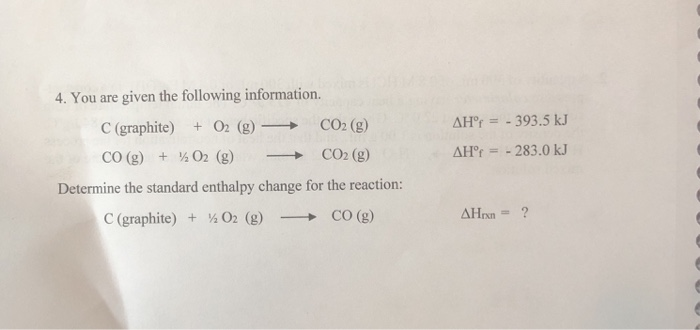4. You are given the following information. C (graphite) + O2(g) — CO2 (g) CO(g) + 02 (g) - CO2 (g) Determine the standard enthalpy change for the reaction: C (graphite) + / O2 (g) - CO (g) AH° = - 393.5 kJ AH° = - 283.0 kJ AHrxn = ?

• ### please help 4. You are given the following information. C (graphite) + O2 (g) - CO2...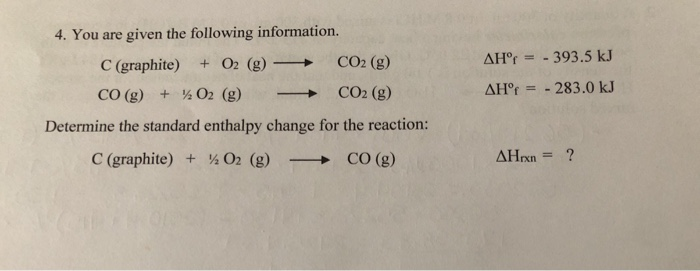please help 4. You are given the following information. C (graphite) + O2 (g) - CO2 (g) CO(g) + 02 (9) - CO2 (g) Determine the standard enthalpy change for the reaction: C (graphite) + 02 (g) - CO(g) AH = - 393.5 kJ AH° = -283.0 kJ AHrxn = ?

• ### 6. Hess's Law. Use the data in Table 1 to calculate AH for the reaction below:...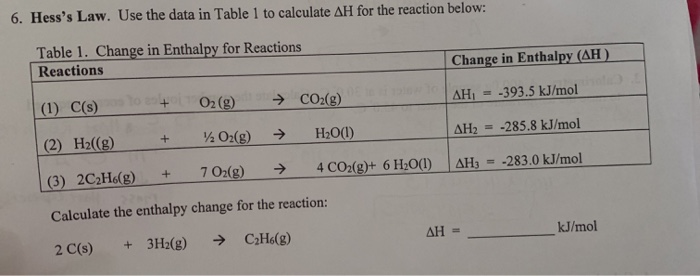6. Hess's Law. Use the data in Table 1 to calculate AH for the reaction below: Table 1. Change in Enthalpy for Reactions Reactions Change in Enthalpy (AH) AH = -393.5 kJ/mol (1) C() (2) Hz((g) + + O2(g) O2(g) → CO2(8) → H2O(l) AH2 = -285.8 kJ/mol (3) 2CH.(g) + 702(g) → 4 CO2(g)+ 6H2O(1) AH = -283.0 kJ/mol Calculate the enthalpy change for the reaction: 2 C(s) + 3H2(g) → CzH6(g) AH = kJ/mol

• ### 6. Hess's Law. Use the data in Table 1 to calculate AH for the reaction below:...6. Hess's Law. Use the data in Table 1 to calculate AH for the reaction below: Table 1. Change in Enthalpy for Reactions Reactions Change in Enthalpy (AH) AH = -393.5 kJ/mol (1) C() (2) Hz((g) + + O2(g) O2(g) → CO2(8) → H2O(l) AH2 = -285.8 kJ/mol (3) 2CH.(g) + 702(g) → 4 CO2(g)+ 6H2O(1) AH = -283.0 kJ/mol Calculate the enthalpy change for the reaction: 2 C(s) + 3H2(g) → CzH6(g) AH = kJ/mol

• ### Given the following equations and AH' values: C(s) + O2(g) + CO2(g) AH = -393.5 kJ...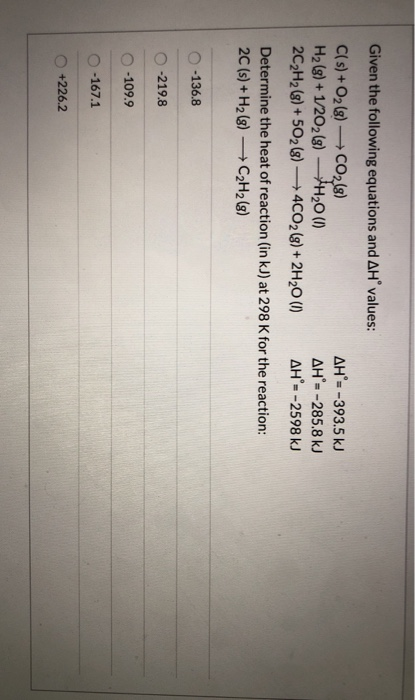Given the following equations and AH' values: C(s) + O2(g) + CO2(g) AH = -393.5 kJ H2(g) + 1/2O2(g) - H20 (1) AH = -285.8 kJ 2C2H2 (8) + 5O2(g) + 4CO2(g) + 2H20 (1) AH'= -2598 kJ Determine the heat of reaction (in kJ) at 298 K for the reaction: 2C(s) + H2(8) C2H2 (8) 0-136.8 0-219.8 0-109.9 O-167.1 +226.2

• ### Given: C(s) + O2(g) ---> CO2(g) ΔH = −393.5 kJ/mol S(s) + O2(g) ---> SO2(g) ΔH...

Given: C(s) + O2(g) ---> CO2(g) ΔH = −393.5 kJ/mol S(s) + O2(g) ---> SO2(g) ΔH = −296.8 kJ/mol C(s) + 2S(s) ---> CS2(ℓ) ΔH = +87.9 kJ/mol A) Calculate the standard enthalpy change for the following reaction CS2(ℓ) + 3O2(g) ---> CO2(g) + 2SO2(g) ΔH° rxn = -1075 kJ/mol B) Using the equation and standard enthalpy change for the reaction (from part A), calculate the amount of heat produced or consumed when 3.2 mol of CS2 reacts with excess...

• ### 7. The enthalpy of combustion of C to CO2 is –393.5 kJ/mol C, and the enthalpy...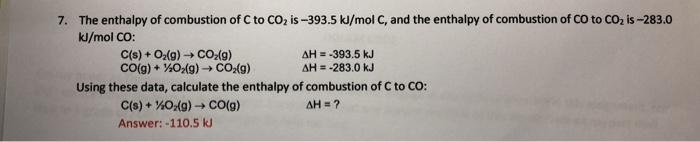7. The enthalpy of combustion of C to CO2 is –393.5 kJ/mol C, and the enthalpy of combustion of Co to CO2 is -283.0 kJ/mol CO: C(s) + O2(g) + CO2(g) AH = -393.5 kJ CO(g) + 102(9) CO2(g) AH = -283.0 kJ Using these data, calculate the enthalpy of combustion of C to CO: C(s) + O2(g) → CO(g) AH = ? Answer: -110.5 kJ